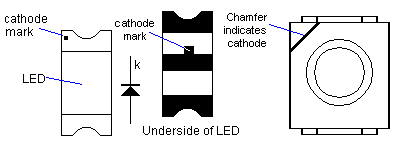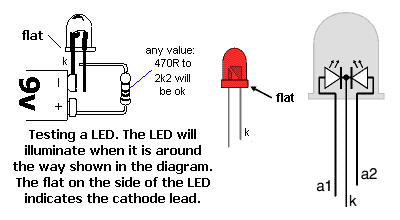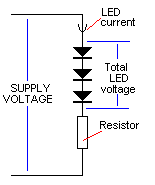HomeArticlesProjectsBlogContact
Articles
Surface Mount LEDsColin Mitchell
Make sure to subscribe to our newsletter and be the first to know the news.
```<script>
var Resistor
var Voltage
var LedVoltage
var Current
var Power

function Process (form) {
if (LedVoltage > 0 && Current > 0 && Voltage > 0) {
Resistor = (Voltage - (LedVoltage)) / (Current / 1000)
if (Resistor <= 0) alert('Supply voltage must be greater than total LED voltages') else {
Resistor = round(Resistor)
standard(Resistor)
Power = (Voltage - (LedVoltage)) * (Voltage - (LedVoltage))
Power = Power / Resistor
Power = round(Power)
form.R.value = Resistor + ' Ohms'
form.P.value = Power + ' Watts'
}
}

function SetLedVolts (X) { LedVoltage = X.value }

function SetI (X) { Current = X.value }

function SetV (X) { Voltage = X.value }

function round (X) {
return Math.round(X * 1000) / 1000
}

function standard (x) {
var r = new Array(10, 11, 12, 13, 15, 16, 18, 20, 22, 24, 27, 30, 33, 36, 39, 43, 47, 51, 56, 62, 68, 75, 82, 91, 100)
LogR = Math.log(x) / Math.log(10)
LogR = Math.floor(LogR)
RA = x / Math.pow(10, LogR - 1)
for (n = 0; n < 25; n++) {
if (RA <= r[n + 1] && RA >= r[n]) {
Ohms = r[n]

if ((r[n] + r[n + 1]) / 2 < RA)
Ohms = r[n + 1]
}
}
Ohms = Ohms * Math.pow(10, LogR - 1)
Resistor = Ohms
K = ' Ohms '
if (Ohms > 999) {
Ohms = Ohms / 1000
K = 'K'
}
if (Ohms > 990) {
Ohms = Ohms / 1000
K = ' Meg'
}
}
</script>
```

The CATHODE lead is always the lead to be identified with ALL LEDs, including surface-mount LEDs.
The cathode of a surface mount LED is very difficult to locate as the device is very small.
There are 3 different identification-marks:

1. A “chamfer” or “cut corner” at one end of the chip
2. A dot on the top left-corner of the device.
3. A mark in the centre of the underside of the chip as shown in the following diagram:The cathode of LEDs in the following shape can be identified by firstly looking for a small protrusion called a “Lead Frame.” It has a hole in it. The lead near it is the ANODE. The opposite lead is the CATHODE.

A “normal” LED (3mm or 5mm) has a small “flat” on the skirt of the LED. This indicates the cathode (k) lead. The cathode lead is the shorter lead.
If both leads have been cut to the same length, and the “flat” is difficult to locate, the LED can be tested by connecting to a battery (6v - 9v) and including a 470R resistor in series.
The LED will illuminate when placed as shown in the diagram. It will not illuminate when around the other way and it will not get damaged during the test.Every LED has a different characteristic voltage drop.
The value depends on the color of the LED, the manufacturer, the batch-number and the type (such as HIGH-Bright, Super-High-Bright etc).
The voltage also changes very slightly according to the current.
When using the LED Resistor Calculator below, the following characteristic voltages can be used:

• Red LED = 1.7v
• Orange LED = 2.1v
• Yellow LED = 2.1v
• Green LED = 2.3v
• High Bright Red = 2v
• White LED = 3.4v
• Super Bright LED - white (6cd) = 3.4v to 4v
• Super Bright LED - blue (3cd) = 3.5v to 4v
• Super Bright LED - red (8cd) = 2.2v to 2.6v
• Super Bright LED - yellow (8cd) = 2.2v to 2.5v
• High Intensity Blue = 4.5v
• InfraRED Laser (27mW) = 1.2v to 1.6v
• Flashing LED (no dropper resistor needed if connected to a supply: 3v - 12v)

(cd = candela 6cd = 6,000mcd)

If you are not sure about a characteristic voltage for a particular LED, use the values above, fit the LED to a circuit and measure the voltage across it.
You can then re-calculate the resistor-value.
Always use 20mA for the allowable current in the calculator below, unless you are informed otherwise. All LEDs will operate for their full operating life (100,000 hrs) @ 20mA.

```<!-- //TODO FIXME: Add led resistor calc -->
```Supply Voltage:

Total LED voltage:

LED Current (mA):

Resistor value:

Power dissipated by resistor:

electronics
writing
PIC-Chips